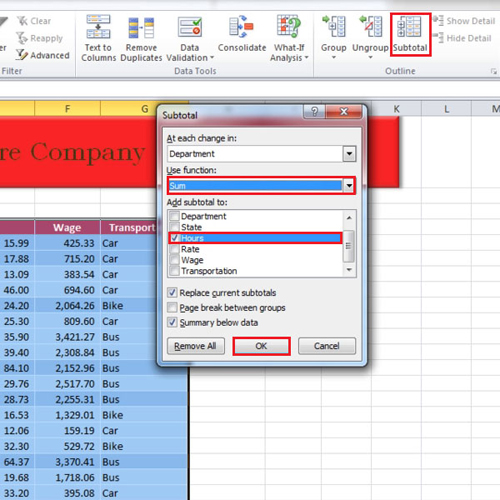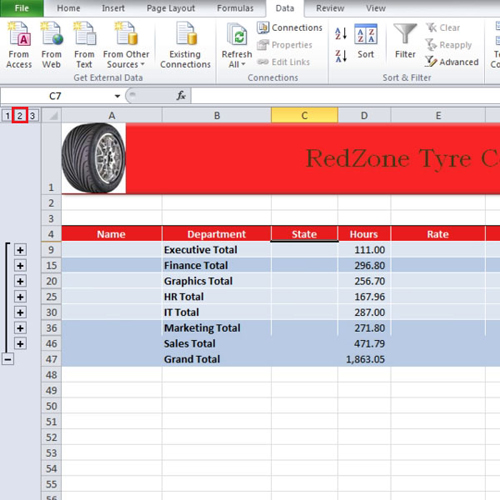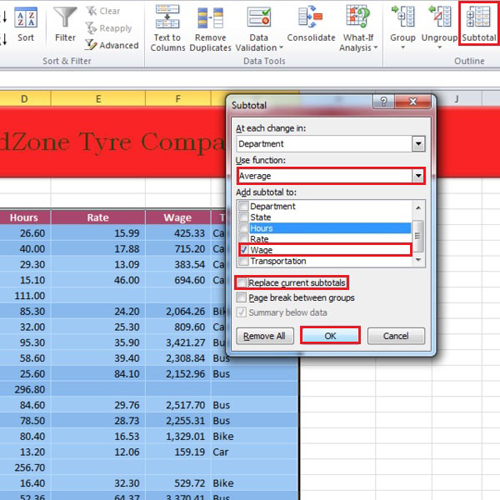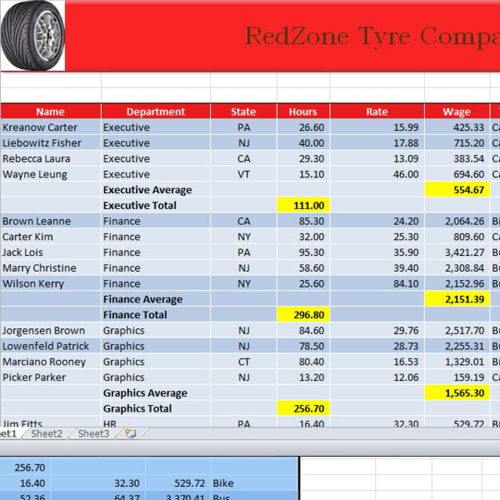## How to insert automatic subtotals in Excel

Excel spreadsheets help users with their calculations and computations. The subtotal feature in Excel allows you to find totals department wise. In this manner, you can compare the individual figures with the grand totals and see each department’s contribution to the grand total. You can make subtotals either using the sum function or working with the average function.
In this tutorial, we will show you how to insert automatic subtotals in Excel.

Step # 1 – Apply Sum Function for Subtotal
First of all, sort the sheet by “department”. Click anywhere within the data and then go to the “data” tab and click on the “subtotal” button. When the “subtotal” box appears, click on the “use function” drop down button and choose the “sum” function. In the tutorial, we want to calculate the “hours”. Thus, we checked the “hours” option and then clicked on the “ok” button.Step # 2 – Subtotals calculated
You will see that below each department, their respective total hours have been calculated. You can then maximize or minimize the ones you want to view or hide, respectively. You can see each department individually or the list of totals along with a grand total for all the departments. In this way, your subtotals in Excel can be computed.Step # 3 – Use the Average function for Subtotals
Next, click on the “subtotal” button again and this time let’s calculate the average “wage”. So this time, we will use the “average” function and select “wage” instead of “hours”.
Since, we don’t want to replace the current subtotals. Therefore, uncheck the “replace current subtotals” option. Now, the average wage will be displayed for each department.Step # 4 – Highlight Subtotals
In order to make the total number of hours and the wage averages prominent or any other sub totals that you have computed, highlight the respective subtotal calculations in Excel.